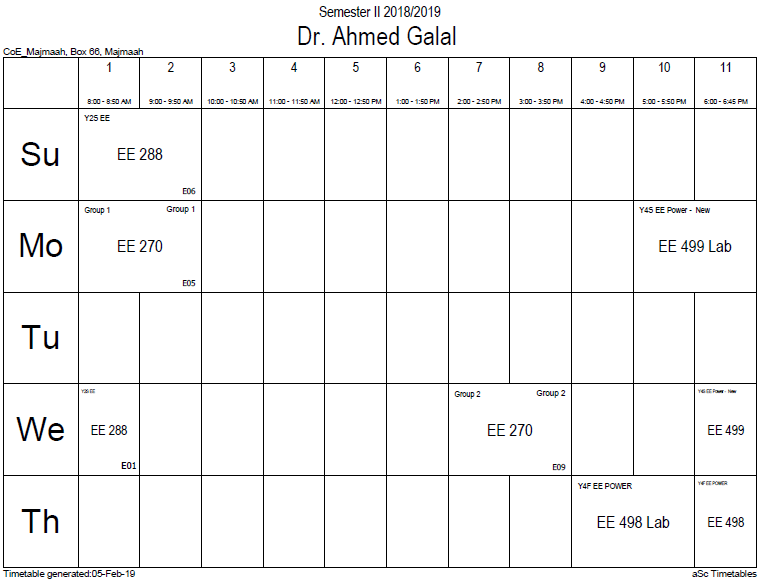## Rotational energy

The rotational energy or angular kinetic energy is the kinetic energy due to the rotation of an object and is part of its total kinetic energy. Looking at rotational energy separately around an object's axis of rotation, one gets the following dependence on the object's moment of inertia:$E_{rotational} = frac{1}{2} I omega^2$

where$omega$ is the Angular velocity$I$ is the moment of inertia around the axis of rotation.$E$ is the kinetic energy.

The mechanical work required for / applied during rotation is the torque times the rotation angle. The instantaneous power of an angularly accelerating body is the torque times the angular velocity. For free-floating (unattached) objects, the axis of rotation is commonly around its center of mass.

Note the close relationship between the result for rotational energy and the energy held by linear (or translational) motion:$E_{translational} = frac{1}{2} m v^2$

In the rotating system, the moment of inertia, I, takes the role of the mass, m, and the angular velocity,$omega$, takes the role of the linear velocity, v. The rotational energy of a rolling cylinder varies from one half of the translational energy (if it is massive) to the same as the translational energy (if it is hollow).

As an example, let us calculate the rotational kinetic energy of the Earth. As the Earth has a period of about 23.93 hours, it has an angular velocity of 7.29×10−5 rad/s. The Earth has a moment of inertia, I = 8.04×1037 kg·m2. Therefore, it has a rotational kinetic energy of 2.138×1029 J.

Part of it can be tapped using tidal power. This creates additional friction of the two global tidal waves, infinitesimally slowing down Earth's angular velocity ω. Due to conservation of angular momentum this process transfers angular momentum to the Moon's orbital motion, increasing its distance from Earth and its orbital period (see tidal locking for a more detailed explanation of this process).

### Office HoursNo office hours### My Timetable### Contactsemail: [email protected]

Phone: 2570

### Welcome

Welcome To Faculty of Engineering### IEEEhttp://www.ieee.org/

/

### Bookmarkshttp://www.utk.edu/research/

http://science.doe.gov/grants/index.asp

http://www1.eere.energy.gov/vehiclesandfuels/

http://www.eere.energy.gov/

### Upcoming Conferences### Engineering quotes### Travel Web Siteshttp://www.hotels.com/

http://www.orbitz.com/

http://www.hotwire.com/us/index.jsp

http://www.kayak.com/

### Blackboardستقام اختبارات الميدتيرم يوم الثلاثاء 26-6-1440

حسب الجدول المعلن بلوحات الاعلان

### Summer trainingThe registration for summer training will start from 5th week of second semesterClass registration week 1

### برنامج التجسير### إحصائية الموقع

عدد الصفحات: 2879

البحوث والمحاضرات: 1280

الزيارات: 101003